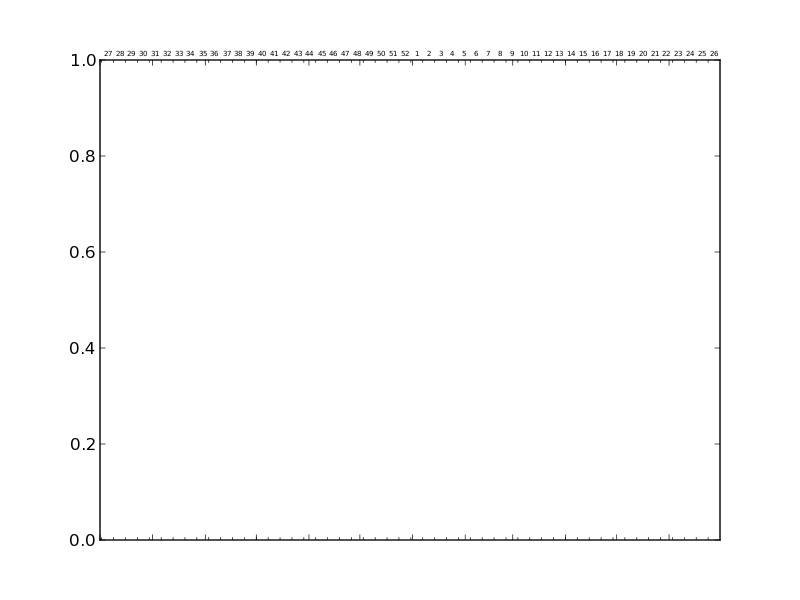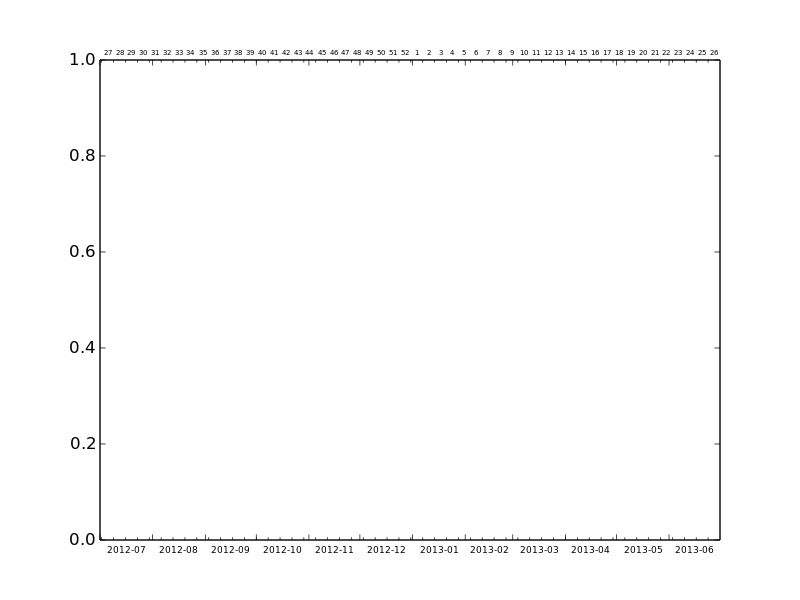# Graph ticks label missing !

Hi,

Following an hardware failure I had to move some matplotlib scripts from
an ancient (but updated) Debian box to a clean RHEL 6 VM. As a result my
matplotlib version changed from 1.2.0 (as present in Debian instable) to
1.2.1 (some of the code wanted matplotlib ≥ 1.0, so I rebuilt an 1.2.1
packaged based on the Fedora 1.2.0 one. 1.3.0-rc2 / 1.3.0git gave me so
many dependencies griefs I gave up on it)

Anyway the result seems to work fine so far, except for a few scores of
time graphs where some tick labels gone missing. Those graphs are supposed
to show weekly data, with:
– ticks on month ends,
– subticks on week ends,
– week numbers in the middle of each week (top) and
– month numbers on the middle of each month (bottom).

With the new installation months numbers got missing.

Here is an extract of the relevant code :

%<---
subplot.set_xticks([dd.monthstart(m) for m in months])
subplot.set_xticks([w.gmticks() for w in weeks], minor=True)
subplot.set_xticklabels([])
plt.xlim(dd.monthstart(min(months)),dd.monthend(max(months)))

subplot1 = subplot.twiny()
mmonths = [m for i,m in enumerate(months)
if operator.mod(i,1+(len(months)-1)/12) == 0]
subplot1.set_xticks([dd.monthmiddle(m) for m in mmonths])
subplot1.set_xticklabels(mmonths)
subplot1.tick_params(labelsize='6.5',labelbottom=True, labeltop=False)
plt.xlim(dd.monthstart(min(months)),dd.monthend(max(months)))

subplot2 = subplot.twiny()
mweeks = [w for i,w in enumerate(weeks)
if operator.mod(i,1+(len(weeks)-1)/53) == 0]
subplot2.set_xticks([dd.weekmiddle(w) for w in mweeks])
subplot2.set_xticklabels([w.iso_week for w in mweeks])
plt.xlim(dd.monthstart(min(months)),dd.monthend(max(months)))

subplot2.xaxis.set_ticks_position('none')
subplot1.xaxis.set_ticks_position('none')
subplot.xaxis.set_ticks_position('both')
%<---

I've tried to move the
subplot1.tick_params(labelsize='6.5',labelbottom=True, labeltop=False)
line to the end of the block, but it seems matplotlib is confusing
subplot1 and subplot2, and that results in the displaying of week numbers
(not month numbers) to the bottom of the plot (and the week numbers
disappear from the top of the graph).

Any idea how to restore the previously working behaviour ?

Regards,

···

--
Nicolas Mailhot

Can you please provide a completely standalone example? The following code has undefined variables etc.

Mike

···

On 07/08/2013 10:49 AM, Nicolas Mailhot wrote:

subplot.set_xticks([dd.monthstart(m) for m in months])
subplot.set_xticks([w.gmticks() for w in weeks], minor=True)
subplot.set_xticklabels([])
plt.xlim(dd.monthstart(min(months)),dd.monthend(max(months)))

subplot1 = subplot.twiny()
mmonths = [m for i,m in enumerate(months)
if operator.mod(i,1+(len(months)-1)/12) == 0]
subplot1.set_xticks([dd.monthmiddle(m) for m in mmonths])
subplot1.set_xticklabels(mmonths)
subplot1.tick_params(labelsize='6.5',labelbottom=True, labeltop=False)
plt.xlim(dd.monthstart(min(months)),dd.monthend(max(months)))

subplot2 = subplot.twiny()
mweeks = [w for i,w in enumerate(weeks)
if operator.mod(i,1+(len(weeks)-1)/53) == 0]
subplot2.set_xticks([dd.weekmiddle(w) for w in mweeks])
subplot2.set_xticklabels([w.iso_week for w in mweeks])
plt.xlim(dd.monthstart(min(months)),dd.monthend(max(months)))

subplot2.xaxis.set_ticks_position('none')
subplot1.xaxis.set_ticks_position('none')
subplot.xaxis.set_ticks_position('both')

Here it is, I'm afraid this testcase intent is less clear than what I
pasted previously (I replaced variables with precomputed values)

As shown in the attached png, the bottom tick labels (month names) are
missing. It worked in matplotlib ≤ 1.2.0

testcase.py (3.33 KB)···

Le Mer 17 juillet 2013 14:56, Michael Droettboom a écrit :

Can you please provide a completely standalone example? The following
code has undefined variables etc.

--
Nicolas Mailhot

Hi,

Can you please provide a completely standalone example? The following
code has undefined variables etc.

Here it is, I'm afraid this testcase intent is less clear than what I
pasted previously (I replaced variables with precomputed values)

As shown in the attached png, the bottom tick labels (month names) are
missing. It worked in matplotlib ≤ 1.2.0

Can you confirm the example was good enough for identifying the problem or
did I forget something again?

Regards,

···

Le Ven 19 juillet 2013 17:47, Nicolas Mailhot a écrit :

Le Mer 17 juillet 2013 14:56, Michael Droettboom a écrit :

--
Nicolas Mailhot

2013/7/19 Nicolas Mailhot <nicolas.mailhot@...547...>:···

Le Mer 17 juillet 2013 14:56, Michael Droettboom a écrit :

Can you please provide a completely standalone example? The following
code has undefined variables etc.

Here it is, I'm afraid this testcase intent is less clear than what I
pasted previously (I replaced variables with precomputed values)

As shown in the attached png, the bottom tick labels (month names) are
missing. It worked in matplotlib ≤ 1.2.0

I can confirm the issue with 1.2.1 but it works with a recent
development version (output attached) so it must have been fixed at
some point.

Goyo

Thank you very much for the data point, I'll try to get 1.3.0 built on RHEL 6

Regards,

···

Le Lun 26 août 2013 18:21, Goyo a écrit :

2013/7/19 Nicolas Mailhot <nicolas.mailhot@...547...>:

Le Mer 17 juillet 2013 14:56, Michael Droettboom a écrit :

Can you please provide a completely standalone example? The following
code has undefined variables etc.

Here it is, I'm afraid this testcase intent is less clear than what I
pasted previously (I replaced variables with precomputed values)

As shown in the attached png, the bottom tick labels (month names) are
missing. It worked in matplotlib ≤ 1.2.0

I can confirm the issue with 1.2.1 but it works with a recent
development version (output attached) so it must have been fixed at
some point.

--
Nicolas Mailhot